## 1、处理丢失数据

• 有两种丢失数据：
• None
• np.nan(NaN)

## 2、两种丢失数据的区别

#### 2.1、None是Python自带的，其类型为Python object。因此，None不能参与到任何计算中。

object类型的运算要比int类型的运算慢得多

``````type(None)
>>>
NoneType
``````

#### 2.2、np.nan（NaN）

np.nan是浮点型，能参与到计算中。但计算结果总是NaN。

``````type(np.nan)
>>>
float
``````

#### 2.3、pandas中的None与NaN

1）pandas中None与np.nan都视作np.nan

``````df = DataFrame({'age':[18,22,34,25,13],
'sex':['男','女','男','男','女']},
index=['小张','小王','小李','小马','小胡'],
columns=['age','sex','hobby'])
``````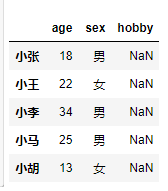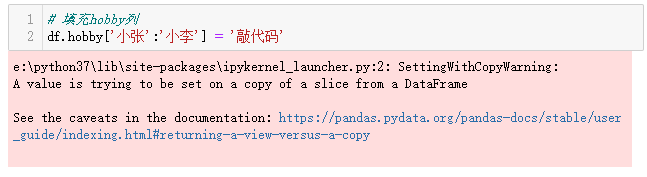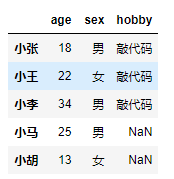#### 2.4、pandas处理空值操作

• isnull
• notnull
• any
• all
• dropna
• fillna
``````小马，小胡的hobby列没有值，需要进行数据清洗。
df.isnull().any(axis=1)#以行为单位
>>>

dtype: bool
``````
``````#查看哪些数据为空值
s = df.isnull().any(axis=1)
df[s]
``````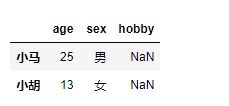``````# 将空的数据进行提取
s1 = df.notnull()
``````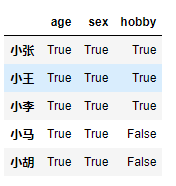``````s2 = s.all(axis=1)
df[s2]
``````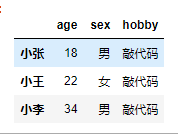notnull()判断数据不为空，所有数据都不为空，才能提取出来，用all(),只要有一个为空用any()。dropna()：高级用法，直接将存在缺失数据的行删除

``````df.dropna(axis=0)
``````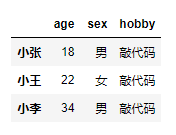``````df.dropna(axis=0,how='any')
``````fillna()：填充丢失数据

​ 填充函数Series/DataFrame

``````#对所有的NaN数据进行替换
df.fillna(value='排BUG')
``````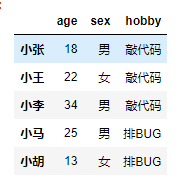``````#用下一行数据进行填充(近邻值)
df.fillna(method='bfill',axis=0)
``````
``````#用上一行数据进行填充(近邻值)
df.fillna(method='ffill',axis=0)
``````